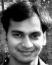# Want to know the level of mathematical questions on the exam ?Hi All,

I just wanted to know the level of mathematical questions on the exam ?

I have already finished questions from http://www.scribd.com/doc/28680971/101-PMP-Sample-Questions-for-the-PMP-Exam.

Any one who recently cleared PMP exam can provide inputs on the level ,is it difficult then above mentioned link or on same level.

### Yes on the scribd, They are good questions

I cleared exam couple of weeks back, The question in scribed are good practice question,. Difficulty level wise I did not find any difficult mathematical questions in the PMP exam. If you know how the calculations are done all questions are easy they are straight forward no tricks in it.

Most difficult part of exams are the situational question, mathematical question are scoring opportunity,### Thanks so much for sharing

Thanks so much for sharing your experience on mathematical question

Welcome

### Depreciation / Final cost of contracts Calculations

There are many depreciation calculations on this site - the only place where I have seen these - are these type of calculations on the PMP exam? Additionally, I have seen calculations where you have to figure out final cost of contracts - are these type on the exam?